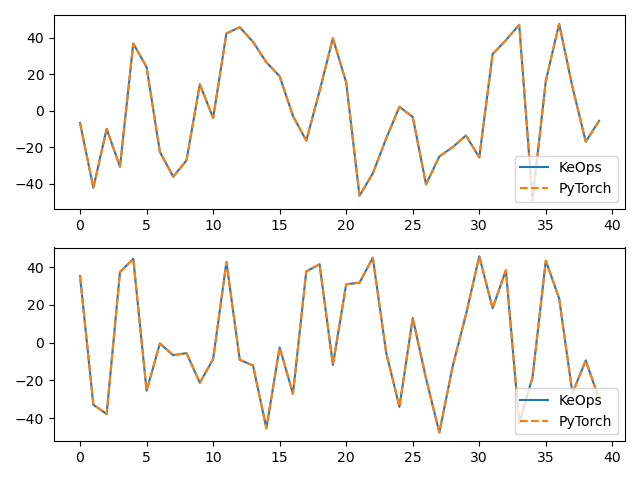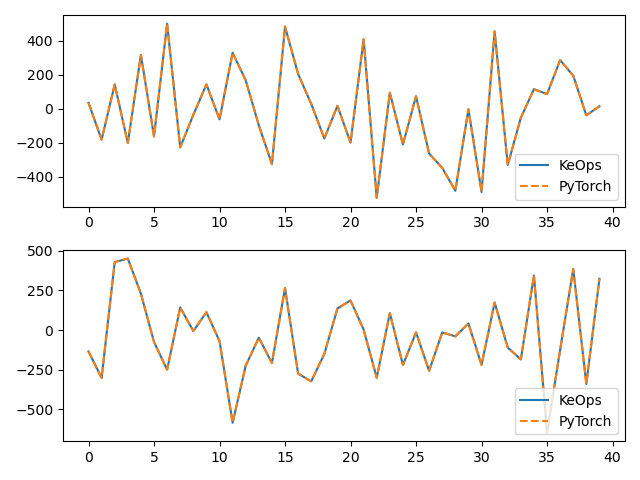# KernelSolve reduction¶

Let’s see how to solve discrete deconvolution problems using the conjugate gradient solver provided by pykeops.torch.KernelSolve.

## Setup¶

Standard imports:

import time

import torch
from matplotlib import pyplot as plt

from pykeops.torch import KernelSolve


Define our dataset:

N = 5000 if torch.cuda.is_available() else 500  # Number of points
D  = 2      # Dimension of the ambient space
Dv = 2      # Dimension of the vectors (= number of linear problems to solve)
sigma = .1  # Radius of our RBF kernel

b = torch.rand(N, Dv)
g = torch.Tensor([ .5 / sigma**2])  # Parameter of the Gaussian RBF kernel

if torch.cuda.is_available():
sync = torch.cuda.synchronize
else:
def sync(): pass


## KeOps kernel¶

Define a Gaussian RBF kernel:

formula = 'Exp(- g * SqDist(x,y)) * b'
aliases = ['x = Vi(' + str(D) + ')',   # First arg:  i-variable of size D
'y = Vj(' + str(D) + ')',   # Second arg: j-variable of size D
'b = Vj(' + str(Dv) + ')',  # Third arg:  j-variable of size Dv
'g = Pm(1)']                # Fourth arg: scalar parameter


Define the inverse kernel operation, with a ridge regularization alpha:

alpha = 0.01
Kinv = KernelSolve(formula, aliases, "b", axis=1)


Note

This operator uses a conjugate gradient solver and assumes that formula defines a symmetric, positive and definite linear reduction with respect to the alias "b" specified trough the third argument.

Apply our solver on arbitrary point clouds:

print("Solving a Gaussian linear system, with {} points in dimension {}.".format(N,D))
sync()
start = time.time()
c = Kinv(x, x, b, g, alpha=alpha)
sync()
end = time.time()
print('Timing (KeOps implementation):', round(end - start, 5), 's')


Out:

Solving a Gaussian linear system, with 5000 points in dimension 2.
Timing (KeOps implementation): 0.30154 s


Compare with a straightforward PyTorch implementation:

sync()
start = time.time()
K_xx = alpha * torch.eye(N) + torch.exp( -torch.sum( (x[:,None,:] - x[None,:,:])**2,dim=2) / (2*sigma**2) )
c_py = torch.solve(b, K_xx)
sync()
end = time.time()
print('Timing (PyTorch implementation):', round(end - start, 5), 's')
print("Relative error = ",(torch.norm(c - c_py) / torch.norm(c_py)).item())

# Plot the results next to each other:
for i in range(Dv):
plt.subplot(Dv, 1, i+1)
plt.plot(   c.cpu().detach().numpy()[:40,i],  '-', label='KeOps')
plt.plot(c_py.cpu().detach().numpy()[:40,i], '--', label='PyTorch')
plt.legend(loc='lower right')
plt.tight_layout() ; plt.show()Out:

Timing (PyTorch implementation): 0.34024 s
Relative error =  0.00031356335966847837
/home/bcharlier/keops/pykeops/examples/pytorch/plot_test_invkernel_torch.py:103: UserWarning: Matplotlib is currently using agg, which is a non-GUI backend, so cannot show the figure.
plt.tight_layout() ; plt.show()


Compare the derivatives:

print("1st order derivative")
e = torch.randn(N,D)
start = time.time()
end = time.time()
print('Timing (KeOps derivative):', round(end - start, 5), 's')
start = time.time()
end = time.time()
print('Timing (PyTorch derivative):', round(end - start, 5), 's')
print("Relative error = ", (torch.norm(u - u_py) / torch.norm(u_py)).item())

# Plot the results next to each other:
for i in range(Dv):
plt.subplot(Dv, 1, i+1)
plt.plot(   u.cpu().detach().numpy()[:40,i],  '-', label='KeOps')
plt.plot(u_py.cpu().detach().numpy()[:40,i], '--', label='PyTorch')
plt.legend(loc='lower right')
plt.tight_layout() ; plt.show()Out:

1st order derivative
Timing (KeOps derivative): 0.37042 s
Timing (PyTorch derivative): 0.36363 s
Relative error =  0.002158492337912321
/home/bcharlier/keops/pykeops/examples/pytorch/plot_test_invkernel_torch.py:131: UserWarning: Matplotlib is currently using agg, which is a non-GUI backend, so cannot show the figure.
plt.tight_layout() ; plt.show()


Total running time of the script: ( 0 minutes 22.639 seconds)

Gallery generated by Sphinx-Gallery